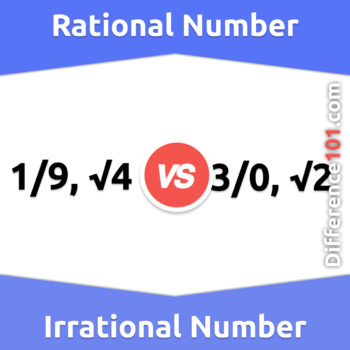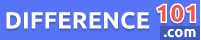# Rational vs. Irrational Numbers: What’s the Difference?

Rational vs. irrational numbers - which one is which? This article will tell you everything you need to know about these two types of numbers, how they differ and how to work with them. Read more here.Rational numbers vs. irrational numbers: Rational numbers can be expressed as fractions. Irrational numbers can’t be expressed as fractions.

As far as both are concerned, numbers are quantities represented by numerals. This arithmetic value can come in the form of a word, symbol, or figure. Numbers are used for measurements, counting, calculations, and labeling, among other things. Examples of numbers include ones that are whole, complex, natural, and real. Integers are also an example.

Rational numbers are written as a ratio of two integers. Irrational numbers cannot be expressed as rational numbers. This means that irrational numbers have decimal expansions that never end or repeat. Examples of irrational numbers include pi (3.14159…) and the square root of 2 (1.414213…). Rational numbers can be easily represented on a number line, but irrational numbers cannot.

Rational numbers may seem similar to irrational ones but are quite different. Rational numbers have an exact value that can be represented through fractions, whereas irrational numbers do not. Irrational numbers also have decimal expansions that go on forever, whereas rational numbers do not.

## What Is A Rational Number?

Rational number definition: a ratio can be defined as a comparison of quantities and is expressed in fraction form. A rational number can be expressed through fractions. For instance, in the fraction p/q, “p” is a numerator while “q” is a denominator. Both are integers. Here, a numerical denominator is natural (non-zero). All integers, fractions (which includes mixed ones), and decimals (including recurring and finite) are rational numbers.

👉  Expression vs. Equation: What is the difference between Expression and Equation?

### Rational Number Examples:

• 1/9 – Here, both the denominator and numerator are integers.
• √16 – In this instance, the number’s square root is 4, with the fraction quotient of 4/1.
• 7 – Written as 7/1. In this case, 7 happens to be the integer quotient of 7 as well as 1.
• 0.3333333333 – The recurring decimals here are rational.
• 0.5 – Expressed as 1/2 or 5/10. The terminating decimals here are rational.

## What Is An Irrational Number?

Irrational number definition: an irrational number simply can’t be expressed as a rational number. In other words, it can’t be expressed as a fraction of two integers (x) and (y). An irrational number’s decimal expansion is neither recurring nor finite – it just goes on forever. Common irrational numbers are π (3.14…) and e (2.718…). Surds are another type of irrational number – they are non-perfect cubes or squares that can’t be reduced further to remove a cube root or square root. For example, √2 is an irrational number because it cannot be expressed as a rational number. However, √4 is a rational number because it can be expressed as 2/2 (two over two).

👉  Anglican vs. Catholic: Everything You Need To Know About The Difference Between Anglican And Catholic

### Irrational Number Examples:

• √2 – This number can’t be simplified. Therefore, it’s irrational.
• 3/0 – Fraction with a denominator of zero, making it irrational.
• √7/5 – In this example, the number given happens to be a fraction. However, it’s not the sole criteria for classifying it as a rational number. In fact, both denominators and numerators must be integers. √7 isn’t an integer. Therefore, the number given is irrational.
• 0.3131131113 – These decimals aren’t recurring or terminating. As such, it can’t be written as a fraction quotient.
• π – 3.14’s decimal value never ends, doesn’t repeat, and does not show a pattern of any kind. As such, pi’s value doesn’t perfectly equate to a fraction. 22/7 happens to be a numerical approximation.
👉  ???? King Crown vs. Queen Crown ????: What is the Difference between King Crown and Queen Crown?

## Primary Distinctions Between Irrational And Rational Numbers

Rational numbers are described as a ratio involving two integers. On the other hand, irrational numbers cannot be written as a ratio of two integers. They include surds (for instance, 2, 5, etc.). A rational number will include those decimals only, which are repeating and finite. On the other hand, irrational numbers have decimal expansions that are non-repetitive, infinite, and lack patterns.

Rational numbers and irrational ones differ in terms of whole numbers, fractions, and perfect squares. A rational number can be a fraction, with both the numerator and denominator being integers. Irrational numbers can’t be written as a fraction, nor can they be written as two whole numbers.

As far as rational numbers go, both denominators and numerators are classified as whole numbers. The denominator doesn’t equal zero. Rational numbers include perfect squares, such as 9 and 25.

## Conclusion

Irrational numbers cannot be expressed as fractions – only in decimal form, as opposed to rational numbers. Additionally, every integer is a rational number, but not every non-integer is irrational. Understanding the difference between rational and irrational numbers is important for anyone working with fractions and decimals.Alex Stantor

Alex Stantor is a Sorbonne University (Paris, France) graduate in Philosophy and Data Analysis. Currently, he is an Author and Researcher at Difference 101, he writes articles/blog posts on topics such as "thinking differently" and "the importance of difference". Alex is a passionate advocate of diversity in the workplace and in companies, and diversity and inclusion in corporate communications. He currently lives in Brooklyn.

We will be happy to hear your thoughts